# Exploratory Analysis II

Data visualization, part 2. Code for quiz 8.

1. Load the R package we will use
``````library(tidyverse)
library(patchwork)
``````

# Question: Modify Slide 51

• Create a plot with `mpg` dataset

• Add points with `geom_point`

• Assign the variable `displ` to the x-axis

• Assign the variable `hwy` to the y-axis

• add `facet_wrap` to split the data into panels based on `manufacturer`

``````ggplot(data = mpg) +
geom_point(aes(x = displ, y = hwy)) +
facet_wrap(facets = vars(manufacturer))
``````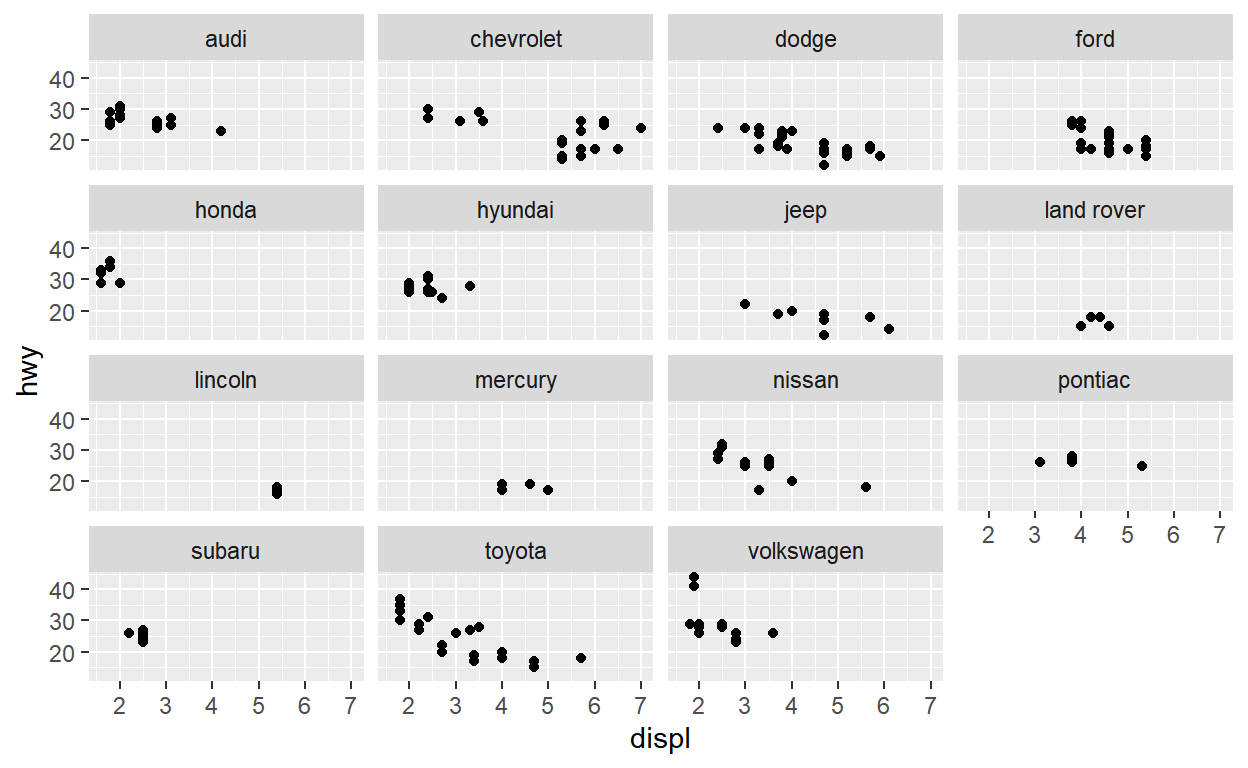# Question: Modify facet-ex-2

• Create a plot with `mpg` dataset

• Add bars with `geom_bar`

• Assign the variable `manufacturer` to the y-axis

• Add `facet_grid` to split data into panels based on `class`

• Let scales vary across columns and let space taken up by panels vary by columns

``````ggplot(mpg) +
geom_bar(aes(y = manufacturer)) +
facet_grid(vars(class), scales = "free_y", space = "free_y")
``````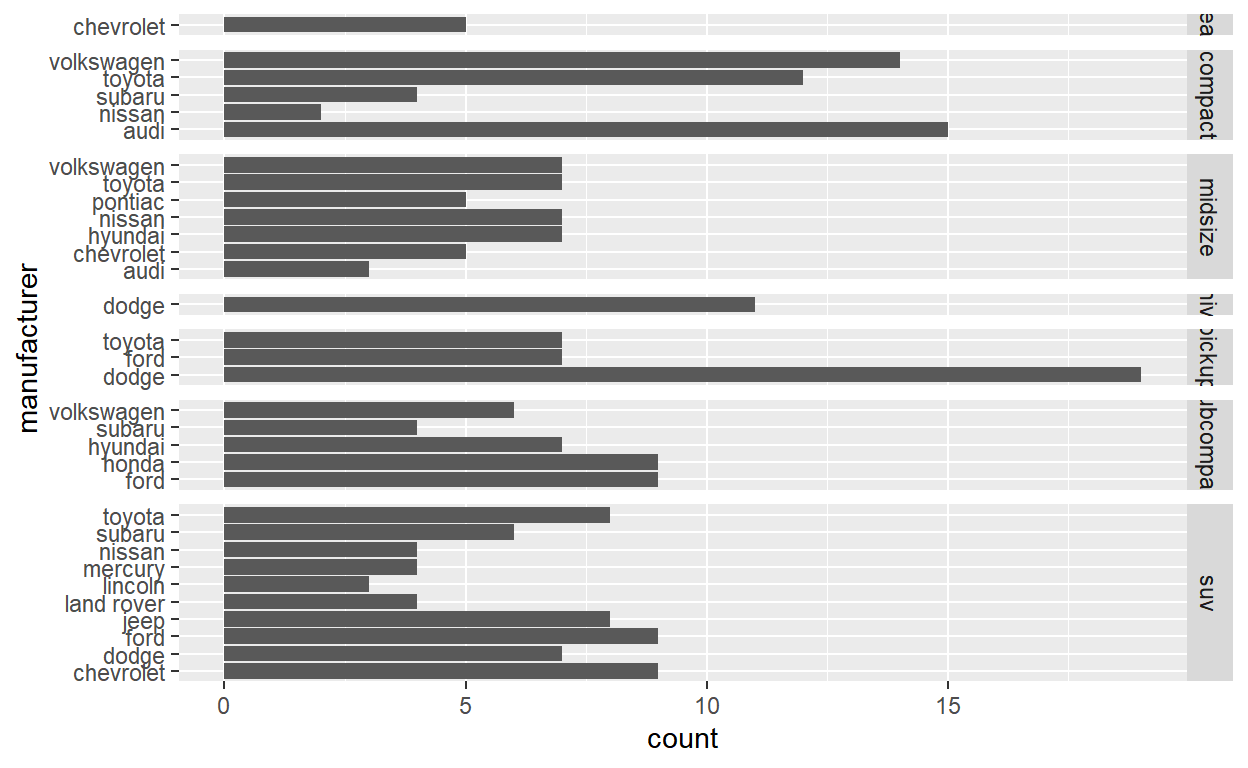# Question: Spend_time

• Read spend_time into `spend_time`
``````spend_time <- read_csv("spend_time.csv")
``````

• Start with `spend_time`

• Extract observations for 2019

• THEN create plot with that data

• Add a barchart with `geom_col`

• Assign `activity` to the x-axis
• Assign `avg_hours` to the y-axis
• Assign `activity` to fill
• Add `scale_y_continuous` with breaks every hour from 0 to 6 hours

• Add `labs` to:

• set `subtitle` to Avg hours per day:2019
• set `x` and `y` to NULL so they won’t be labeled
• Assign the output to `p1`

• Display `p1`

``````p1 <- spend_time %>% filter(year == 2019) %>%
ggplot()+
geom_col(aes(x = activity, y = avg_hours, fill = activity)) +
scale_y_continuous(breaks = seq(0,6, by = 1)) +
labs(subtitle = "Avg hours per day: 2019", x = NULL, y = NULL)

p1
``````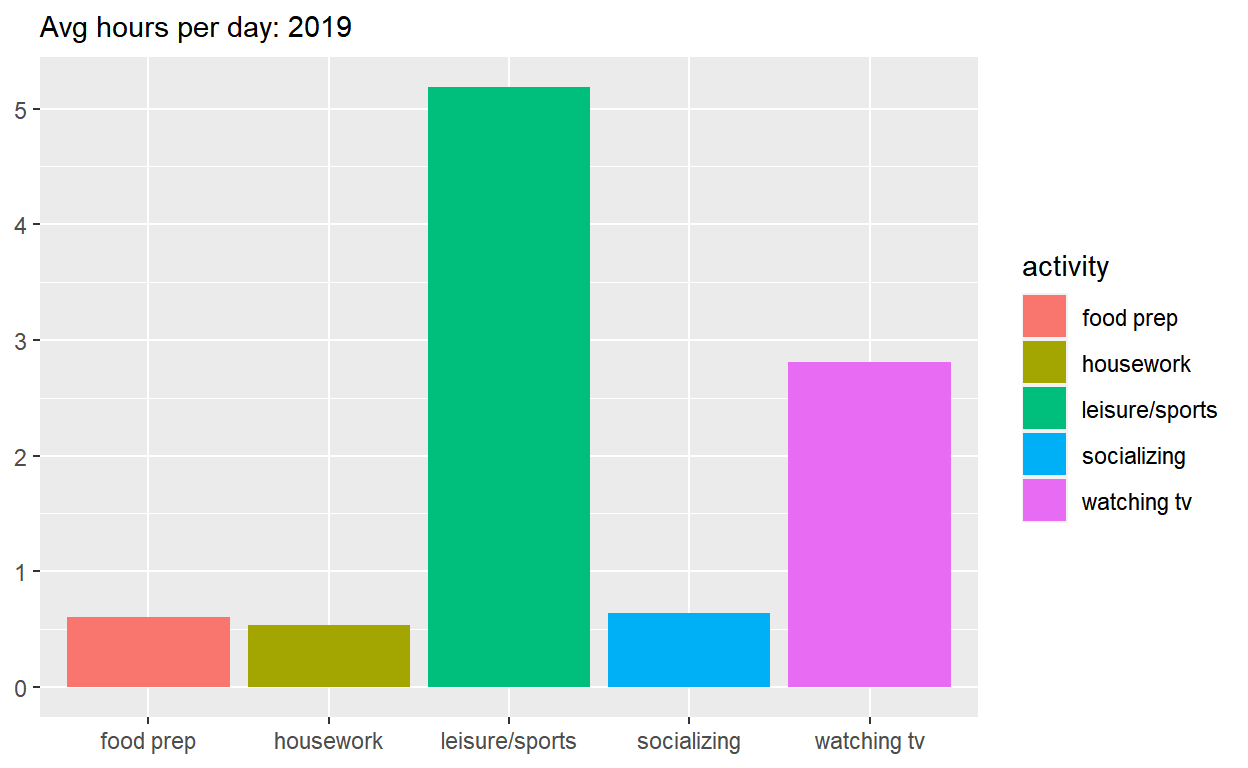• Start with `spend_time`

• THEN create plot with it

• Add a barchart with `geom_col`

• Assign `year` to the x-axis
• Assign `avg_hours` to the y-axis
• Assign `activity` to fill
• Add `labs` to:

• Set subtitle to Avg hours per day: 2010-2019
• Set x and y to NULL so they won’t be labeled
• Assign the output to `p2`

• Display `p2`

``````p2 <- spend_time %>%
ggplot() +
geom_col(aes(x = year, y = avg_hours, fill = activity)) +
labs(subtitle = "Avg hours per day: 2010-2019", x = NULL, y = NULL)

p2
``````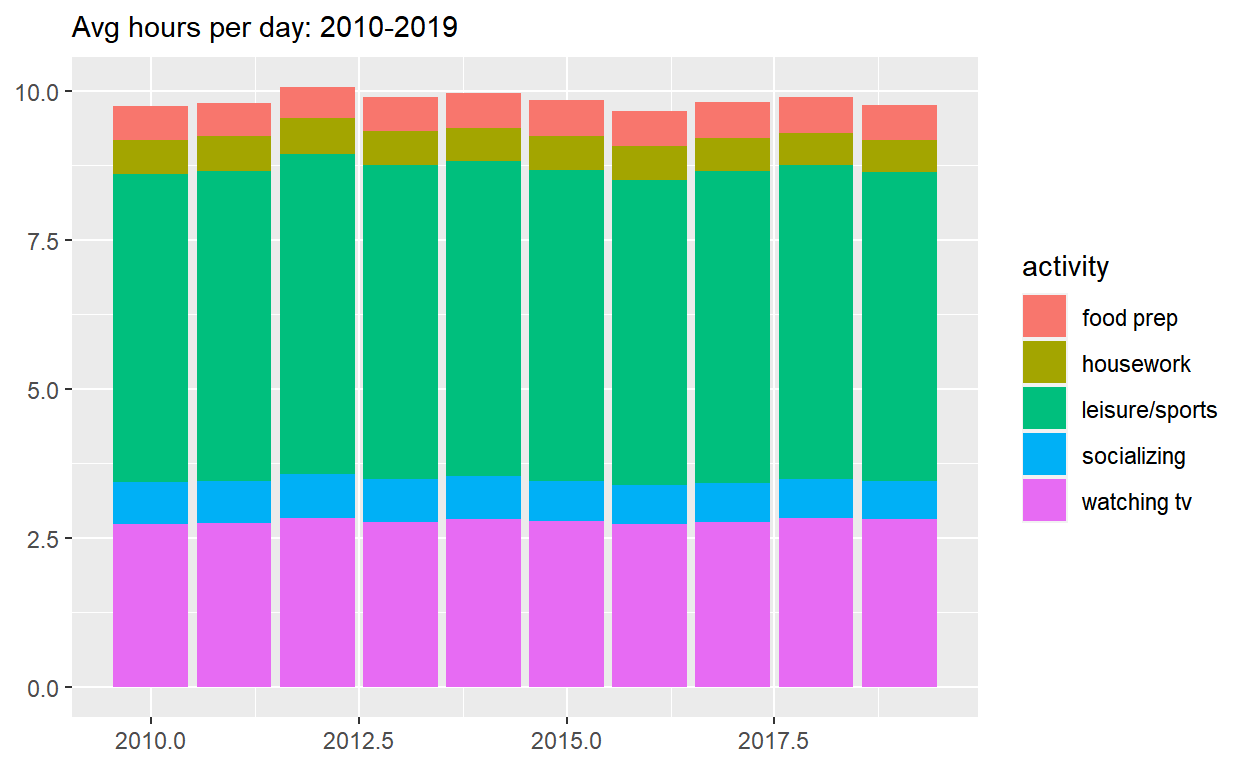• Use `patchwork` to display `p1` on top of `p2`

• Assign the output to `p_all`

• Display `p_all`

``````p_all <- (p1/p2)

p_all
``````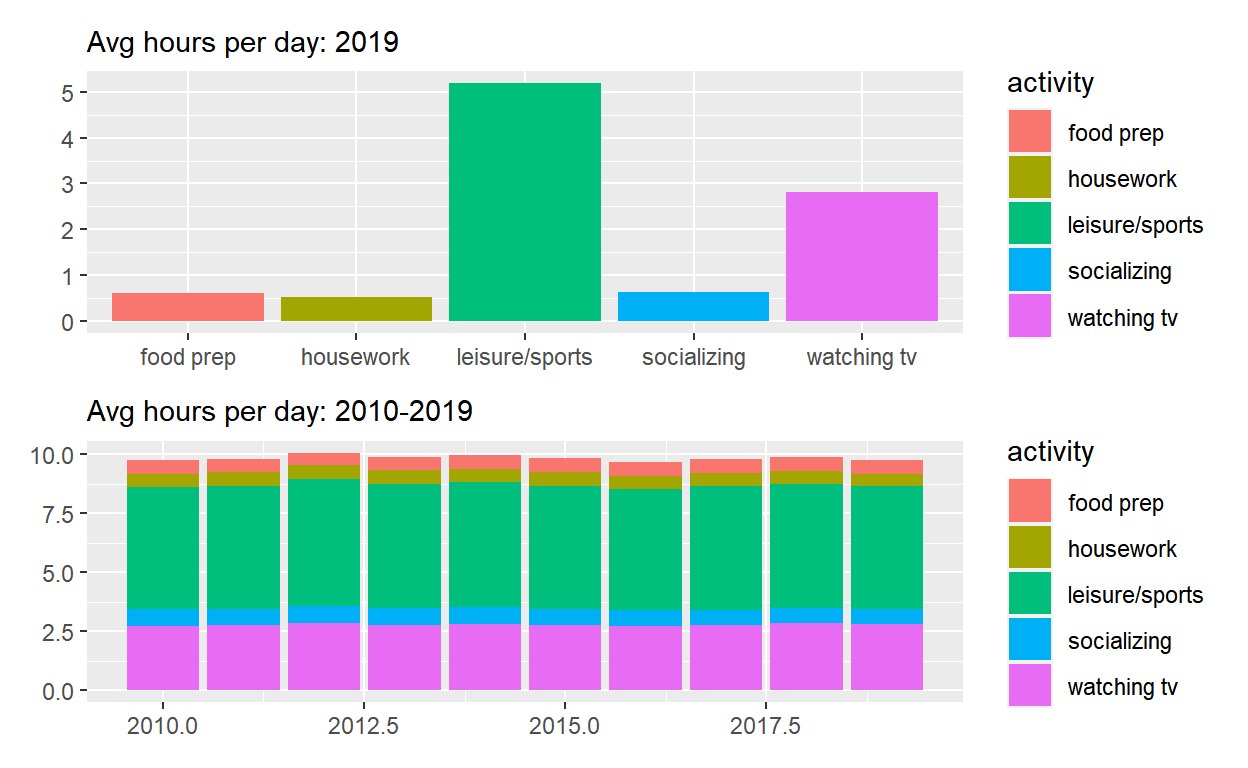• Start with `p_all`

• And set `legend.position` to “none” to get rid of the legend

• Assign the output to `p_all_no_legend`

• Display `p_all_no_legend`

``````p_all_no_legend <- p_all & theme(legend.position = "none")

p_all_no_legend
``````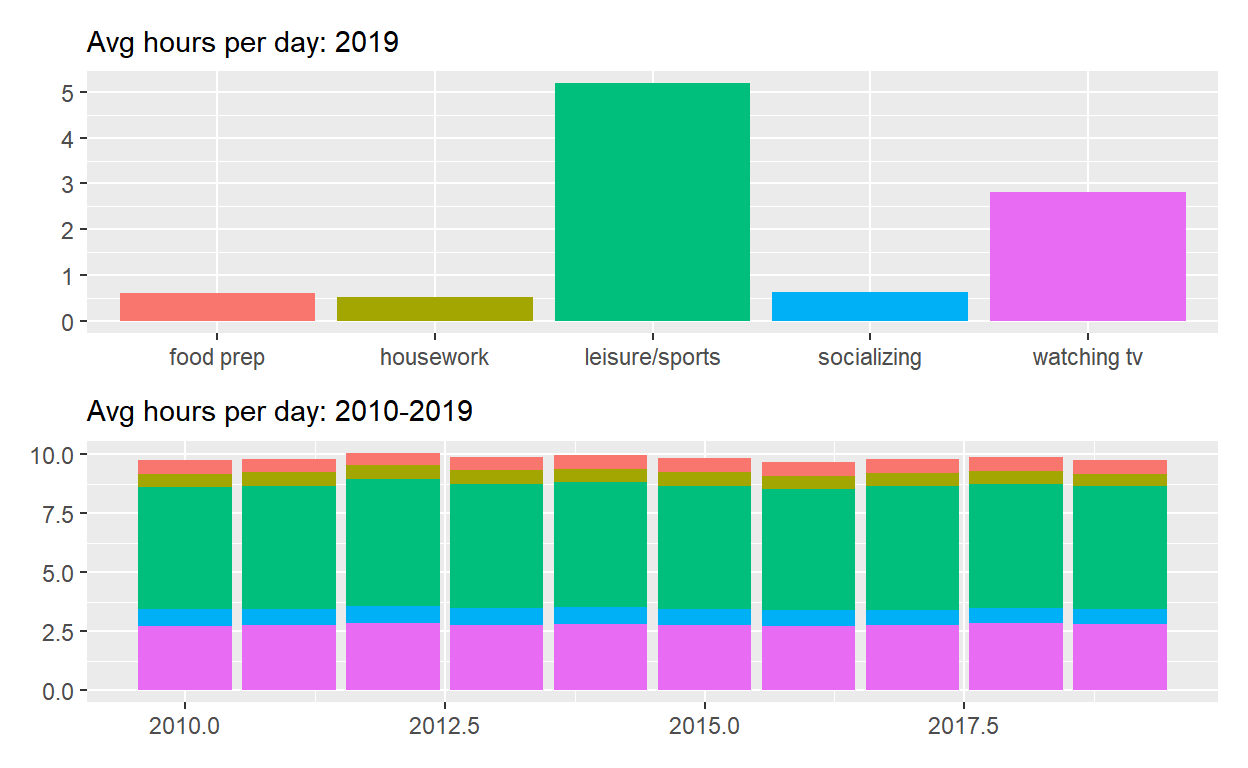• Start with `p_all_no_legend`

• Add `plot_annotation` set

• Add `title` to “How much time Americans spent on selected activities”

• `Caption` to “Source: American Time of Use Survey, https://data.bls.gov/cgi-bin/surveymost?tu

``````p_all_no_legend +
plot_annotation(title = "How much time Americans spent on selected activities",
caption = "Source: American Time of Use Survey, https://data.bls.gov/cgi-bin/surveymost?tu ")
``````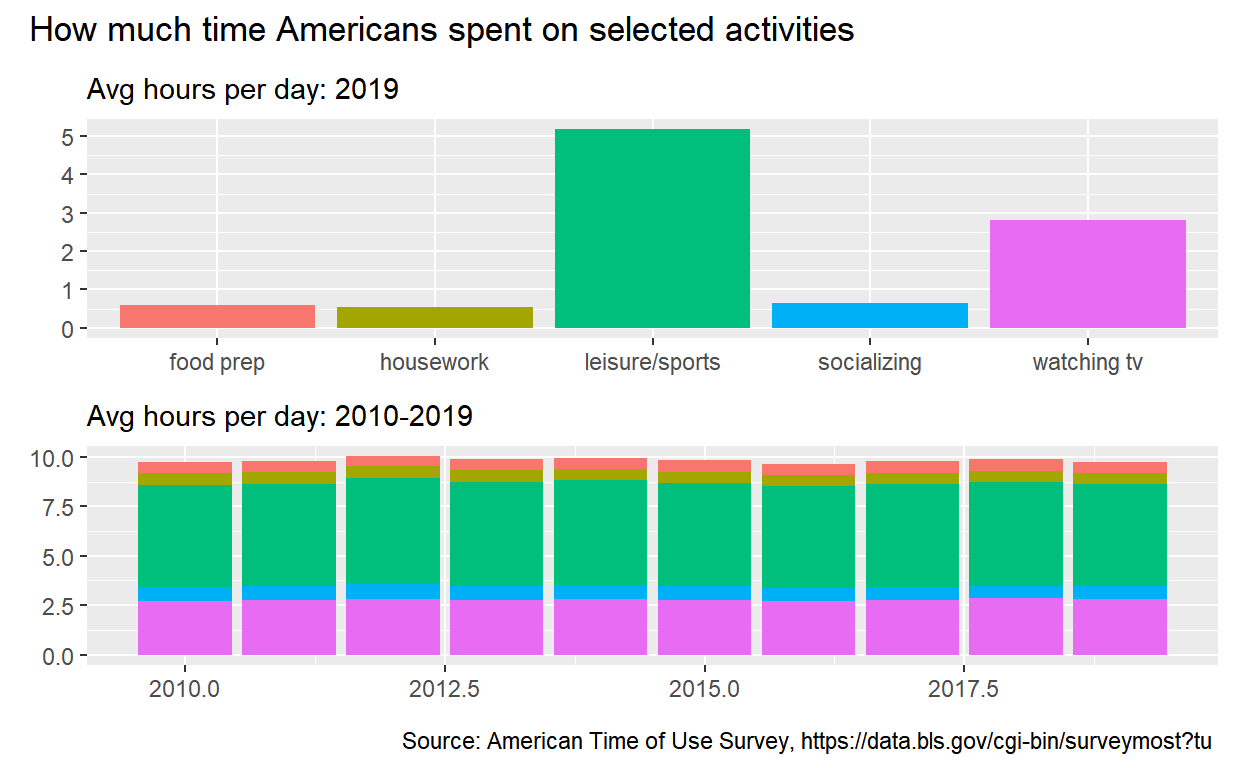# Question: Patchwork 2

• Start with `spend_time`

• Extract observations for leisure/sports

• THEN create plot with data

• Add points with `geom_point`

• Assign `year` to the x-axis
• Assign `avg_hours` to the y-axis
• Add line with `geom_smooth`

• Assign `year` to the x-axis
• Assign `avg_hours` to the y-axis
• Add breaks on for every year on x-axis with `scale_x_continuous`

• Add `labs` to:

• Set `subtitle` to Avg hours per day: leisure/sports
• Set `x` and `y` to NULL so x and y axes won’t be labeled
• Assign the output to `p4`

• Display `p4`

``````p4 <- spend_time %>%
filter(activity == "leisure/sports") %>%
ggplot() +
geom_point(aes(x = year, y = avg_hours)) +
geom_smooth(aes(x = year, y = avg_hours)) +
scale_x_continuous(breaks = seq(2010, 2019, by = 1)) +
labs(subtitle = "Avg hours per day: leisure/sports", x = NULL, y = NULL)

p4
``````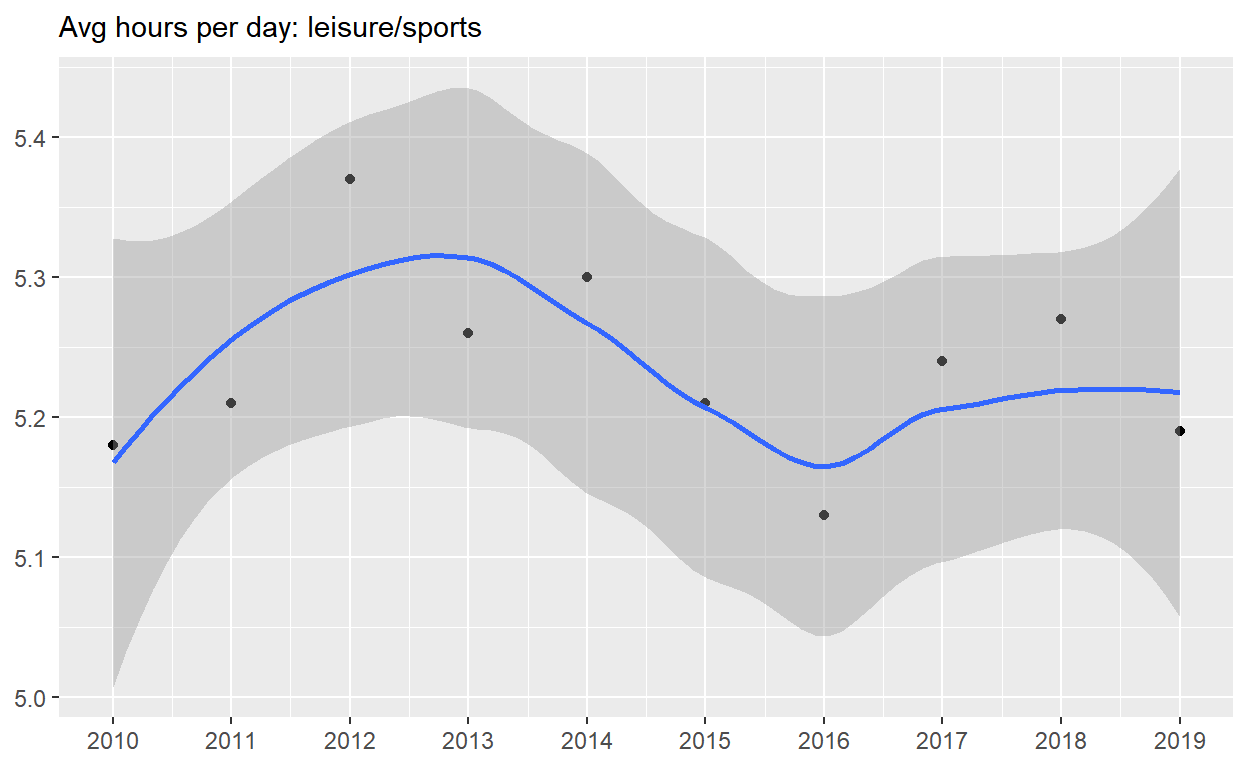• Start with `p4`

• Add `coord_cartesian` to change range on y axis to 0 to 6

• Assign the output to `p5`

• Display `p5`

``````p5 <- p4 + coord_cartesian(ylim = c(0,6))

p5
``````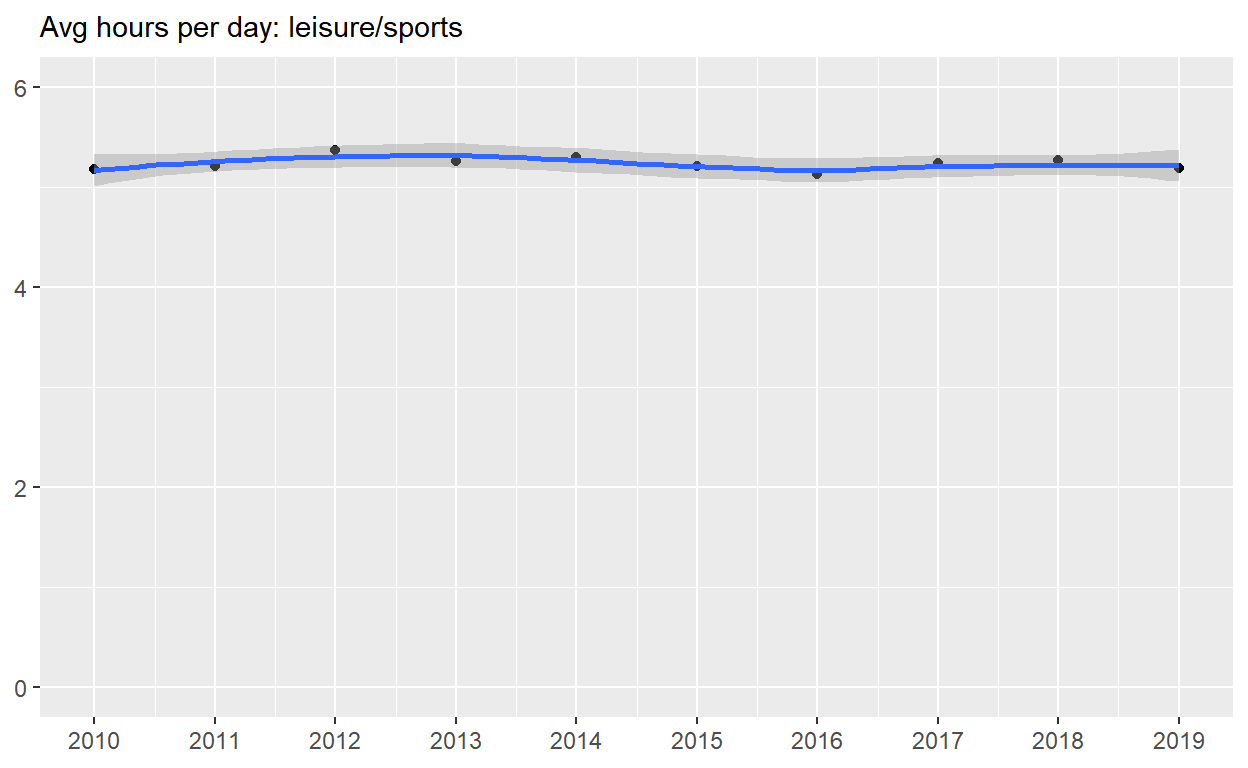Start with `spend_time`

• Create a plot with data

• Add points with `geom_point`

• Assign `year` to the x-axis

• Assign `avg_hours` to the y-axis

• Assign `activity` to color

• Assign `activity` to group

• Add line with `geom_smooth`

• Assign `year` to the x-axis

• Assign `avg_hours` to the y-axis

• Assign `activity` to color

• Assign `activity` to group

• Add breaks for every year on the x-axis with `scale_x_continuous`

• Add `coord_cartesian` to change range on y-axis from 0 to 6

• Add `labs` to:

• set x and y to NULL so they wont be labeled
• Assign the output to `p6`

• Display `p6`

``````p6 <- spend_time %>%
ggplot() +
geom_point(aes(x = year, y = avg_hours, color = activity, group = activity)) +
geom_smooth(aes(x = year, y = avg_hours, color = activity, group = activity)) +
scale_x_continuous(breaks = seq(2010, 2019, by = 1)) +
coord_cartesian(ylim = c(0,6)) +
labs(x = NULL, y = NULL)

p6
``````• Use `patchwork` to display p4, p5 and p6
``````(p4/p5) / p6
``````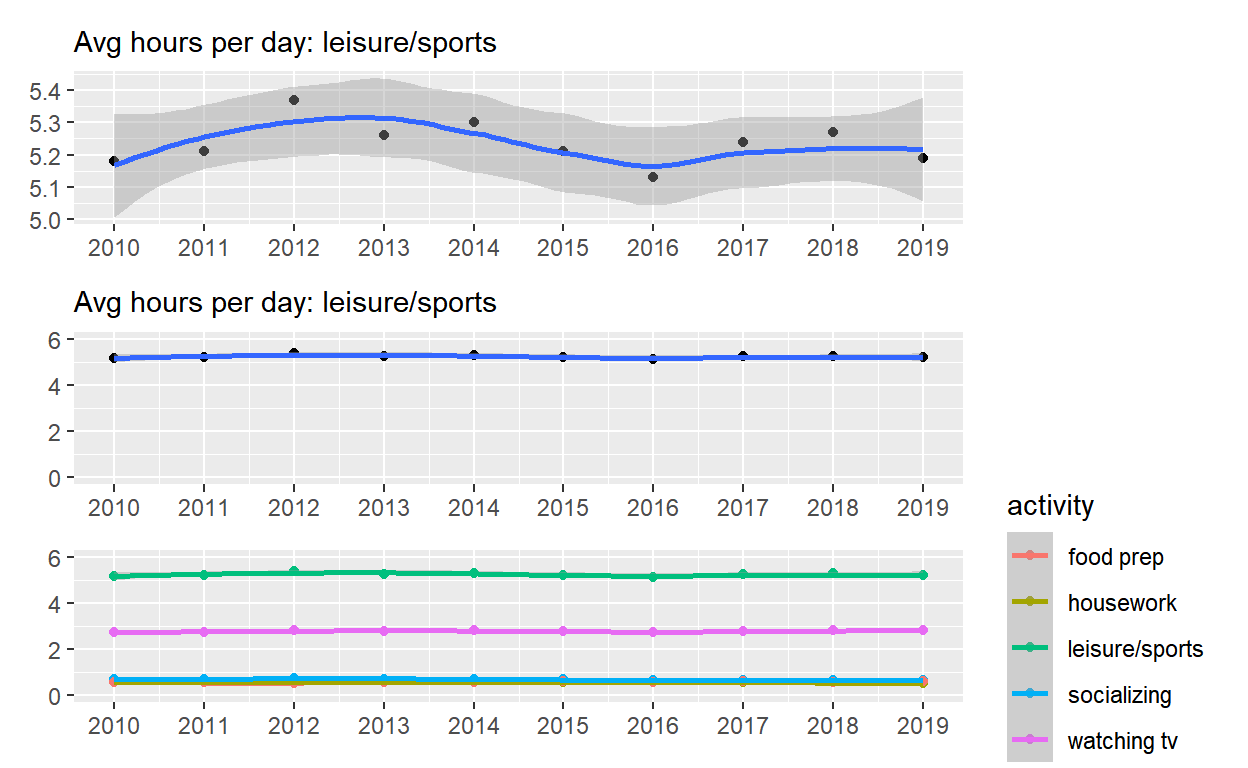``````ggsave(filename = "preview.png", path = here::here("_posts", "2022-03-25-exploratory-analysis-ii"))
``````